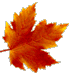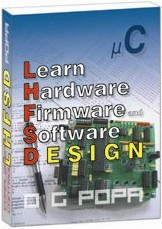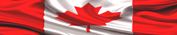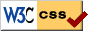HOME PAGE --------------- HELP+ » e-Design Notes --- » Switches, R, F » Resistors » Capacitors » Inductors » Diodes » Transistors --- » Potential Diff » Op Amps » Analog Filters --- » Boolean Algebra » Logic Gates » Flip-Flops » Logic ICs --- » Auto Injectors ---------------# ELECTRONIC DESIGN NOTES - BOOLEAN ALGEBRAAlgebra--such a beautiful name! Algebra was brought to us by the Arab migratory people in the seventh century. More specifically, the name "Algebra" is derived from Al-Jabar [Al-Jabar means "reunion" in Persian] the title of a book written by the great mathematician Muhammad Ibn Musa Al-Khwarizmi in 820. However, Algebra was first used during the great--and the most mysterious--Sumerian Civilization about 7000 years ago!

Now, the true beauty about Algebra is, it is a logic mind-game! In fact, there are two logic systems that could help us gain the most in terms of brain-power:
1. Analytic Plane Geometry
2. Algebra

Both of them are branches of Mathematics, and we hope we shall start one good sunny day to write a few "Math Reference Notes" in this site.

Now, hardware, firmware and software are related, because they all work with the same mathematical model: the binary code--this is, a special interpretation model built on the Base 2 numerical system. Another common name used instead of binary code is "digital". Frankly, we have absolutely no idea why this name, "digits", sounded that impressive during the golden years 1950s and 1960s. Anyway, digital/logic electronics means electronic applications of the Base 2 numerical system. Therefore, in digital electronics we work with only two states:
1. one
2. zero"One" in hardware is a saturated transistor; "zero" represents its cutoff state [or backwards, for negative logic]. In firmware one is one set bit, and zero is the bit cleared. Of course, in software things behave exactly as they do in firmware, because software is translated into firmware and, eventually, into machine code.

The power of the Base 2 Numerical System is enormous, particularly because it is the simplest, though complete, mathematical model. Although the Base 10 numerical system we currently use in our life appears to be more complex, and elegant, Base 2 is far more powerful. In fact the Base 2 numerical system is the one and only UNIVERSAL MATHEMATICAL MODEL. Wow!

In order to present Boolean Algebra in this page, the following simplified structure was employed:

1. Basic notions in Boolean Algebra
2. Boolean Theorems
3. Equivalent switching circuits

NOTE

The basic notions highlighted in this page are related to a few electronic design topics presented in the first part, Hardware Design, of
LEARN HARDWARE FIRMWARE AND SOFTWARE DESIGN.

1. BASIC NOTIONS IN BOOLEAN ALGEBRA

Boolean Algebra
is a mathematical model developed for the TRUE and FALSE states by Mr. George Boole (1815-1864). Unfortunately, throughout his life Mr. Boole's work was not much appreciated by ordinary engineers; he was "discovered" very late, in 1930, when Boolean Algebra started being used to simulate switching circuits.

Boolean Algebra is a particular application of the Base 2 numerical system, therefore it also deals with 2 states:
1. True, commonly related to the mathematical "one"
2. False, commonly related to the mathematical "zero"

Only two operations have been defined for Boolean Algebra; they are:
1. Addition (+)
2. Multiplication (*)

ATTENTION
The addition and multiplication operations of Boolean Algebra ARE NOT mathematical addition and multiplication. These two logic operations use the signs (+) and (*) in order to facilitate understanding Boolean Algebra, but never forget that they are not the mathematical addition and multiplication. The rules governing Boolean addition and multiplication follow next.

The sign "=" used in Boolean Algebra IS NOT the mathematical "=". In Boolean Algebra "=" means "may be substituted with/for" not "equal to".

1.1 BASIC BOOLEAN OPERATIONS

A + 0 = A
A * 0 = 0

A + 1 = 1
A * 1 = A

A * A = 0
A + A = 1

A * A = A + A = A

ATTENTION
The meaning of A is, complement of A. For example: if A is 0, then A is one; however, A may be 1, and its complement A is zero in this case. Another example is: 1 = 0, and 0 = 1.1.2 ASSOCIATIVE RULES
"Associative" in mathematics is the quality of "regrouping operands/terms".

1.2.1 BOOLEAN MULTIPLICATION

A * (B * C) = (A * B) * C

1.2.2 BOOLEAN ADDITION

A + (B + C) = (A + B) + C

1.3 COMMUTATIVE RULES
"Commutative" is a term used in mathematics with the meaning: "it may change places".

For example:

A + B = B + A
The above expression is valid in Boolean Algebra, and its meaning is: the Boolean operation of addition is commutative.

A * B = B * A
The above expression it is also valid in Boolean Algebra, and its meaning is: the Boolean operation of multiplication is commutative.

1.4. DISTRIBUTIVE RULES
The "distributive" term is used to mark particular properties of mathematical operations. In our case, Boolean Algebra, the meaning is, the operation of addition (+) is distributive when it is related to the operation of multiplication (*), and backwards.

A * (B + C) = A * B + A * C
A + B * C = (A + B) * (A + C)

The above relations are very important, and they are used a lot.1.5. SIMILARITY OF BOOLEAN ALGEBRA TO THE MATHEMATICAL LOGIC "AND" AND "OR"
The AND logic operation used in firmware/software is expressed in Boolean Algebra as:

A (AND) B = A * B

The OR logic operation is expressed in Boolean Algebra as:

A (OR) B = A + B

ATTENTION
The last two logic equations above exemplify perfectly clear that the Boolean (+) and (*) operations are not the mathematical addition and multiplication.

2. BOOLEAN THEOREMS

The English mathematician Mr. Augustus de Morgan (1806 - 1871)--he worked together with Mr. George Boole--is one of the founders of the Boolean Algebra. He left us a few nice theorems as follows.

2.1. DE MORGAN THEOREMS

A * B = A + B
A + B = A * B

2.2. OTHER BOOLEAN THEOREMS

A * (A + B) = A
A + A * B = A

A * (A + B) = A * B (also noted as AB for simplification)
A + A * B = A + B

A * B + A * B = A
(A + B) * (A + B) = A

2.3. INCLUDED TERM AND INCLUDED FACTOR THEOREMS

A * B + B * C + C * A = A * B + C * A
(A + B) * (B + C) * (C + A) = (A + B) * (C +A)

The Boolean theorems presented in this page are the most common constructions used to simplify Boolean logic. In the next section you will see a few practical implementations of the Boolean logic functions.3. EQUIVALENT SWITCHING HARDWARE CIRCUITS

The simple switching circuits presented further down should be sufficient to understand practical implementation in hardware of Boolean Algebra. For clarity, we have attached the correspondent firmware/software logic equivalent formulas.

 BOOLEAN EQUIVALENT OF SWITCHING CIRCUITS CIRCUIT DESCRIPTIONFig 1 : Function LED  LED = A * B  (in Boolean hardware) is equivalent to: LED = A AND B (in firmware logic)Fig 2 : Function LED LED = A + B  (in Boolean hardware) LED = A OR B (in firmware logic)Fig 3 : Function LED LED = A * B * (C + D)  (in Boolean hardware) LED = A AND B AND (C OR D) (in firmware logic)Fig 4 : Function LED  LED = A * D + B * C (in Boolean hardware) LED = (A AND D) OR (B AND C) (in firmware logic)Fig 5 : Function LED  LED = A * (B + C) (in Boolean hardware) LED = A AND (B OR C) (in firmware logic)Fig 6 : Function LED  LED = A * B + A * B (in Boolean hardware) LED = (A AND B) OR [(NOT A) AND (NOT B)] (in firmware logic)Take control of the extraordinary IT Revolution with Learn Hardware Firmware and Software Design--the easy way!» LINK TO "LOGICALLY STRUCTURED ENGLISH GRAMMAR"
» BACK TO TOPSend your comments regarding this page using support@corollarytheorems.com, noi@editcc.com, or cus@2agraph.com
Page last updated on: July 25, 2018
© SC Complement Control SRL. All rights reserved.Site pages valid according to W3CStylesheets pages valid according to W3C